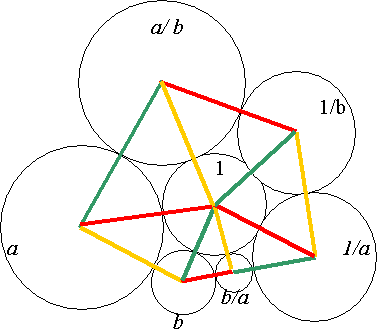You may also likeBaby Circle

A small circle fits between two touching circles so that all three circles touch each other and have a common tangent? What is the exact radius of the smallest circle?Fixing It

A and B are two fixed points on a circle and RS is a variable diamater. What is the locus of the intersection P of AR and BS?Kissing

Two perpendicular lines are tangential to two identical circles that touch. What is the largest circle that can be placed in between the two lines and the two circles and how would you construct it?

Flower

Age 16 to 18Challenge Level

You are given a circle of radius 1 unit and two circles of radius $a$ and $b$ which touch each other and also touch the unit circle. Prove that you can always draw a 'flower' with six petals (as in the sketch) with the unit circle in the middle, and six circles around it having radii $a$, $b$, $b/a$, $1/a$, $1/b$ and $a/b$, such that each outer circle touches the unit circle and the two circles on either side of it.

[Note: this diagram is not drawn accurately. Drawing your own more accurate diagram may help you to do the question.]